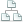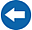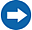Home >Tips >Excel 2016 from Scratch >Custom number formats BSite map

# Excel 2016 from Scratch Exercise Custom Number Formats B

## An Excel tutorial by Peter KalmstromIn this exercise in the Excel 2016 from Scratch series, Peter Kalmstrom shows how to set custom number formats in Excel.

Text cannot be calculated, so a custom number format is useful when you want to add some text, for example a sort, in a cell. If you use a customer number format for that, Excel will stil consider the figures in the cell a number, and it will be possible to use it in a calculation.

As an example Peter uses ferries that take passengers around in the Stockholm archipelago. For the calculation of number of tours and passengers, it is more clear to have the sorts "pass" (for passanger) and "tour" in the cells where the number is given.

### Exercise

If you want to try the exercise yourself, please download the Excel file Peter uses in the demo!

### Content

This is what Peter shows in the demo below:

• How to name a cell to use it as an absolute reference.
• How to multiply the values of two cells.
• How to divide the values of two cells.
• How to change a decimal value into percentage.
• How to reduce the number of decimals for a range of cells.
• How to apply Cell Styles to differentiate cells with input values from cells with calculated values.
• How to create a custom number format.
Peter uses Excel 2016 for his demo, but the Excel calculation functions are the same for earlier versions of Excel.